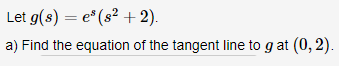# Let g(s) e (s2 +2) a) Find the equation of the tangent line to g at (0, 2)

Question

Find the equation of the tangent line.help_outlineImage TranscriptioncloseLet g(s) e (s2 +2) a) Find the equation of the tangent line to g at (0, 2) fullscreen

### Want to see this answer and more?

Experts are waiting 24/7 to provide step-by-step solutions in as fast as 30 minutes!*

*Response times vary by subject and question complexity. Median response time is 34 minutes and may be longer for new subjects.
Tagged in
MathCalculus

### Other